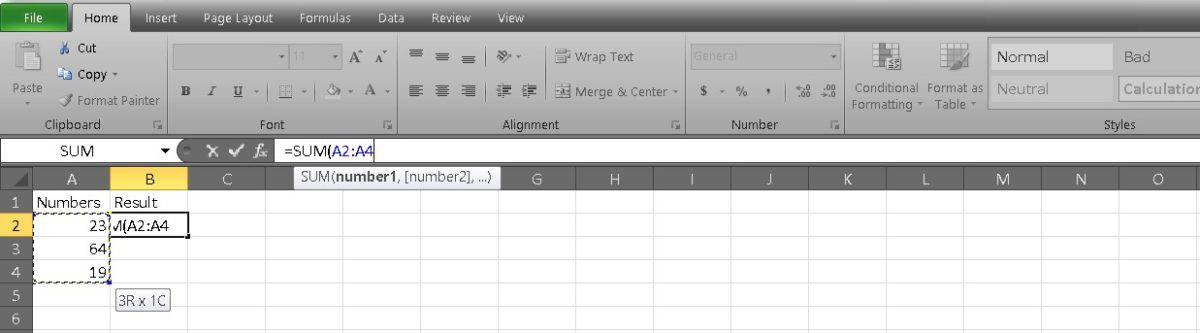# How to add cells in Excel

The most essential features in Excel are often the simplest ones. You’ll find that almost every project requires some degree of math. In this case, we’re talking about the essential skill of adding cells. However, there are quite a few different ways to accomplish your goal. Here are two quick ways to add cells in Excel.

To add cells in Excel, use the SUM() function, entering the cells you want to add inside the brackets. Press Enter to see your results.

## How to add cells in Excel

The most basic way to add cells in Excel is to write a formula.

Start with an equals sign (=) followed by your cells separated by a plus sign (+). In our example, this reads as =A2+A3+A4.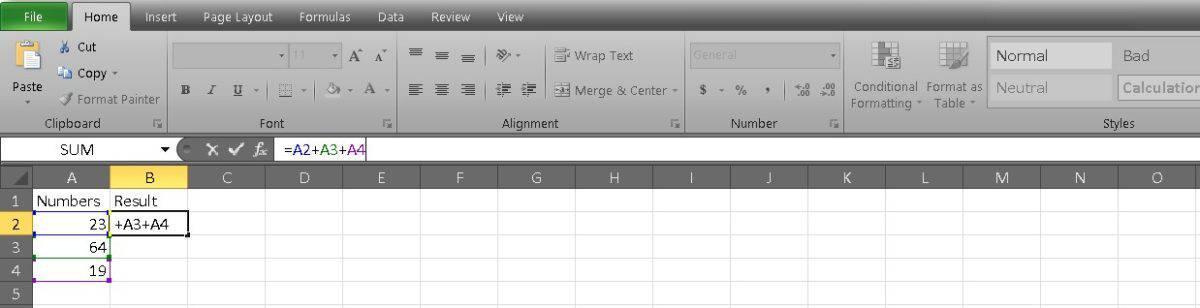Press Enter to get your result.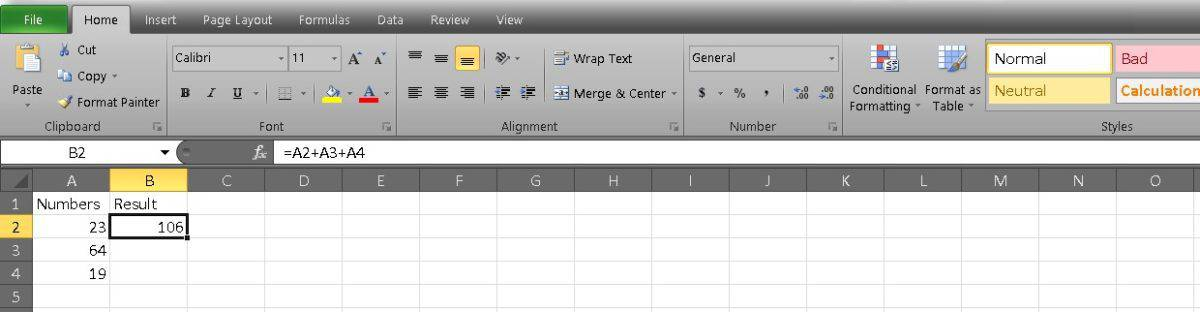This method is easiest if you add just a few cells and don’t mind typing them. However, you’ll probably find that using the SUM function to add long strings of cells is faster.

Start with the equals sign again, as this is how all formulas start. Then type SUM( to create your function.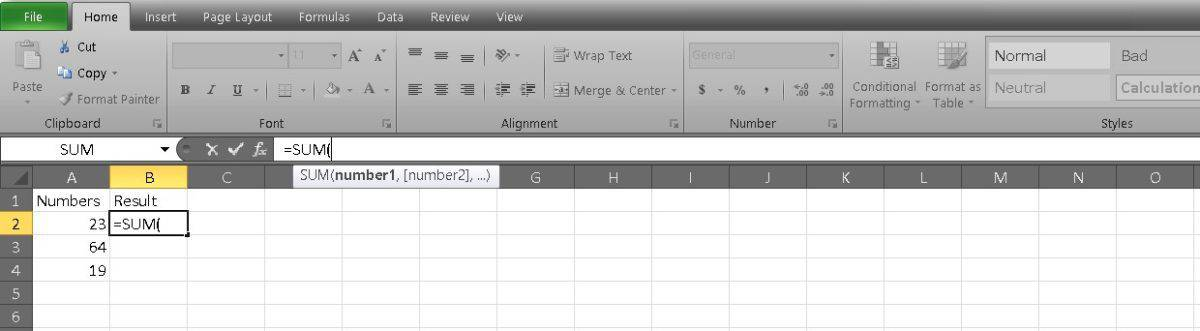Now you can type in the cells you want to add and divide them with commas. This would read as such: =SUM(A2,A3,A4).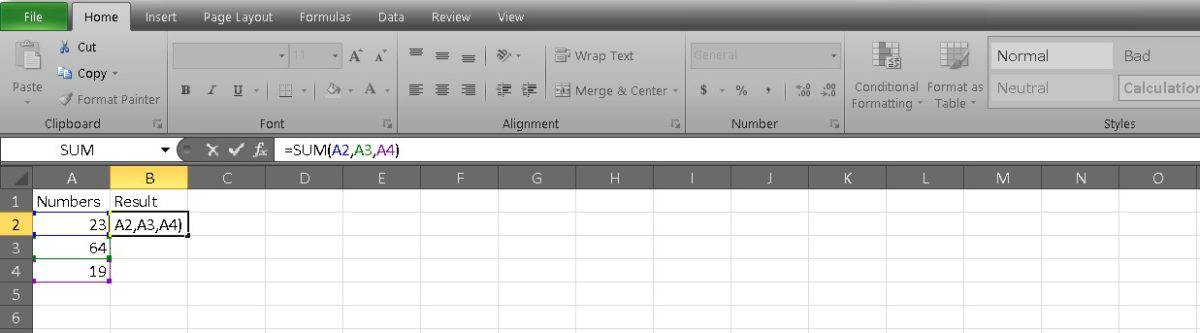If you’re adding a string of unbroken cells, you can separate them in your equation with a colon. This is easier if you have a long list because you can enter just the first and last cells. Our example looks like this: =SUM(A2:A4).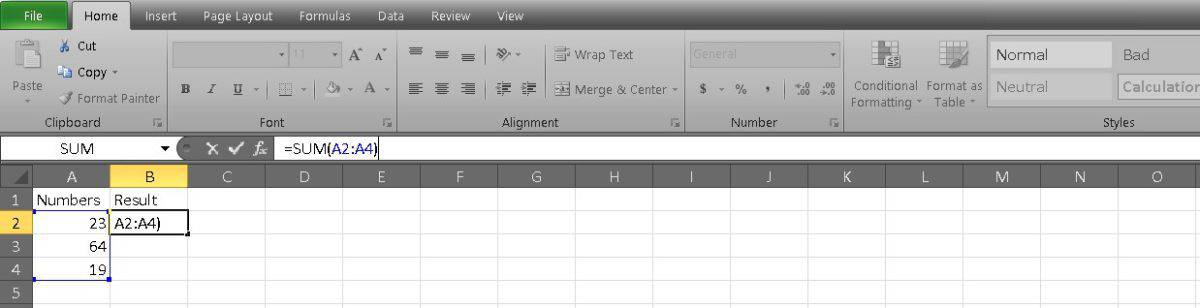A third way you can populate your formula is by clicking and dragging. After you’ve written =SUM, you can select the first cell you want to add and drag it to the last cell. Excel will do the rest and separate it with a colon.